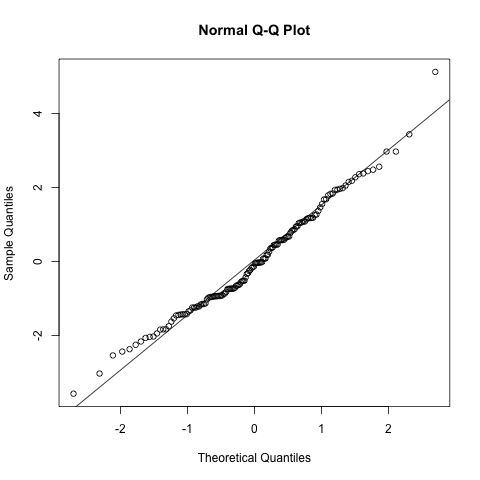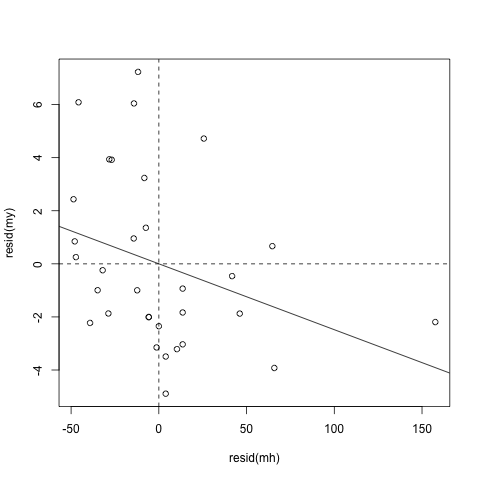# Overview

anova(object_1, object_2)
Compare a submodel with an outer model and produce an analysis of variance table.
coef(object)
Extract the regression coefficient (matrix). Long form: coefficients(object).
deviance(object)
Residual sum of squares, weighted if appropriate.
formula(object)
Extract the model formula.
plot(object)
Produce four plots, showing residuals, fitted values and some diagnostics.
predict(object, newdata=data.frame)
The data frame supplied must have variables specified with the same labels as the original. The value is a vector or matrix of predicted values corresponding to the determining variable values in data.frame.
print(object)
Print a concise version of the object. Most often used implicitly.
residuals(object)
Extract the (matrix of) residuals, weighted as appropriate. Short form: resid(object).
step(object)
Select a suitable model by adding or dropping terms and preserving hierarchies. The model with the smallest value of AIC (Akaike’s An Information Criterion) discovered in the stepwise search is returned.
summary(object)
Print a comprehensive summary of the results of the regression analysis.
vcov(object)
Returns the variance-covariance matrix of the main parameters of a fitted model object.

# Fit a model with no intercept howto

lm(y ~ 0 + x)

You can use this feature for Parameterization, which makes the resulting coefficients independent.

coef(lm(Hwt ~  Bwt + Sex, data = MASS::cats))
coef(lm(Hwt ~ 0 + Bwt + Sex, data = MASS::cats))
(Intercept)         Bwt        SexM
-0.41495263  4.07576892 -0.08209684
Bwt       SexF       SexM
4.0757689 -0.4149526 -0.4970495


# Interpret coefficients of categorical variables in lmhowto

Consider the following model: $Y = \beta_0 + \beta_1 x_1 + \beta_2 x_2 + \beta_3 x_1 x_2 + \epsilon$

mpg_hp_add = lm(mpg ~ hp + am + hp:am, data = mtcars)
coef(mpg_hp_add)
  (Intercept)            hp            am         hp:am
26.6248478696 -0.0591369818  5.2176533777  0.0004028907


In this case, am is a categorical variable, which determine the observation is automatic or manual.

(Intercept)
is the estimated average mpg for a car with an automatic transmission and 0 hp.
(Intercept) + am
is the estimated average mpg for a car with a manual transmission and 0 hp.
am
is the estimated difference in average mpg for cars with manual transmissions as compared to those with automatic transmission, for any hp.
hp
is the estimated change in average mpg for an increase in one hp, for either transmission types.
hp:am
is the estimated difference in the change in average mpg for an increase in disp, for a car of a certain hp (or vice versa).

# Calculate confidence intervals for model parameters howto

fit <- lm(100/mpg ~ disp + hp + wt + am, data = mtcars)
confint(fit)
confint(fit, "wt")
                   2.5 %      97.5 %
(Intercept) -0.774822875 2.256118188
disp        -0.002867999 0.008273849
hp          -0.001400580 0.011949674
wt           0.380088737 1.622517536
am          -0.614677730 0.926307310
2.5 %   97.5 %
wt 0.3800887 1.622518


# Draw Fitted versus Residuals Plot howtoplot

m = lm(Hwt ~ Bwt, data = MASS::cats)
plot(fitted(m), resid(m))
abline(h = 0)Linearity
Residuals should be centered at 0.
Equal Variance
Variances are similar across fitted values.

# Draw Normal QQ Plot howtoplot

• QQ stands for Quantile-Quantile.
• X axis for theoretical quantiles, Y axis for sample quantiles.

As for checking normality of errors:

• X axis goes to the normal distribution quantiles
• Y axis goes to the residuals of the model.
m = lm(Hwt ~ Bwt, data = MASS::cats)
qqnorm(resid(m))
qqline(resid(m))Explanatory function
qqplot = function(e) {
n = length(e)
# For n = 10, this vector contains quantiles of [0.05 0.15 0.25 ... 0.95]
normal_quantiles = qnorm(((1:n - 0.5) / n))
# plot theoretical verus observed quantiles
plot(normal_quantiles, sort(e))

# calculate line through the 1st and 3rd quartiles
slope     = (quantile(e, 0.75) - quantile(e, 0.25)) / (qnorm(0.75) - qnorm(0.25))
intercept = quantile(e, 0.25) - slope * qnorm(0.25)
# add to existing plot
abline(intercept, slope, lty = 2, lwd = 2, col = "dodgerblue")
}

# Perform BP test howto

• Test homoscedasticity. (whether or not the error is equally distributed)
m = lm(Hwt ~ Bwt, data = MASS::cats)
lmtest::bptest(m)

studentized Breusch-Pagan test

data:  m
BP = 9.5294, df = 1, p-value = 0.002022



# Perform Shapiro-Wilk Test howto

• Unlike other test functions, shapiro.test accepts a vector instead of a lm object.
shapiro.test(rnorm(25))

Shapiro-Wilk normality test

data:  rnorm(25)
W = 0.91987, p-value = 0.0509



# Calculate Leverage howto

m = lm(mpg ~ hp, data = mtcars)
unname(hatvalues(m))
  0.04048627 0.04048627 0.05102911 0.04048627 0.03675069 0.04317538
 0.09757509 0.08046517 0.04958291 0.03510034 0.03510034 0.03886509
 0.03886509 0.03886509 0.05458370 0.06327290 0.07888001 0.07592585
 0.09277415 0.07704010 0.04819161 0.03132530 0.03132530 0.09757509
 0.03675069 0.07592585 0.05253020 0.03903750 0.12568847 0.03675069
 0.27459288 0.04099664


# Calculate Standardized Residual howto

Calculates: $r_i = \frac{e_i}{s_e\sqrt{1 - h_i}}$

m = lm(mpg ~ hp, data = mtcars)
unname(rstandard(m))
  -0.42118619 -0.42118619 -0.25341558 -0.31547671  0.14271781 -1.27952886
  0.24990523 -0.39648860 -0.21698865 -0.66062513 -1.02957426 -0.37436332
 -0.13671767 -0.69122419 -1.52076121 -1.34530901  0.07920301  1.83232949
  1.04609436  2.21923491 -0.52556608 -1.14798677 -1.22689309 -0.02259947
  0.27459819  0.45893182  0.56112724  2.11548290  1.02806065  0.40647857
  2.35785298 -0.33359639


# Find influential observations

Calculates and test the value heuristically:

\begin{aligned} D_i &= \frac{1}{p}r_i^2\frac{h_i}{1 - h_i}\\ D_i &> \frac{4}{n} \quad \text{(when it's true, the observation is influential)} \end{aligned}
m = lm(mpg ~ hp, data = mtcars)
unname(cooks.distance(m) > 4 / nrow(mtcars))
  FALSE FALSE FALSE FALSE FALSE FALSE FALSE FALSE FALSE FALSE FALSE FALSE
 FALSE FALSE FALSE FALSE FALSE  TRUE FALSE  TRUE FALSE FALSE FALSE FALSE
 FALSE FALSE FALSE FALSE FALSE FALSE  TRUE FALSE


# Calculate Variance Inflation Factors howto

You can use car::vif(model). It appears that many packages include this kind of functions.

m = lm(mpg ~ disp + hp, data = mtcars)
car::vif(m)
    disp       hp
2.670938 2.670938


Also, we can calculate it manually:

m = lm(mpg ~ disp + hp, data = mtcars)
md = lm(disp ~ hp, data = mtcars)
mh = lm(hp ~ disp, data = mtcars)
c(1 / (1 - summary(md)$r.squared), 1 / (1 - summary(mh)$r.squared))
 2.670938 2.670938


If the VIF of a parameter is larger than 5, heuristically it can be considered to be somewhat collinear to other variables.

# Calculate Partial Correlation Coefficient howto

my = lm(mpg ~ disp, data = mtcars)
mh = lm(hp ~ disp, data = mtcars)
cor(resid(mh), resid(my))
 -0.3258012


When this value is large, we can think that the unexplained portion of the candidate predictor, hp in the example above, and that of the response are highly correlated. This means that there is no significant collinearity between the candidate predictor and the existing predictors, disp in this case. So, adding the candidate predictor is of benefit.

We can also visualize this relationship:

plot(resid(my) ~ resid(mh))
abline(h = 0, lty = 2)
abline(v = 0, lty = 2)
abline(lm(resid(my) ~ resid(mh)))# Perform a model selection with exhaustive search howto

• Use leaps::regsubsets(formula, data = data)
• This returns a list of best models of each size.

# Perform a model selection with stepwise search based on AIC/BIC howto

# Backward AIC
selected_model = step(full_model, direction = "backward")
# Backward BIC
selected_model = step(full_model, direction = "backward", k = log(n))
# Forward AIC
selected_model = step(start_model, scope = formula, direction = "forward")
# Both AIC
selected_model = step(start_model, scope = formula, direction = "both")

Note that it is not available to use . in scope. To use . for the scope, you can pass a list to scope as follows:

selected_model = step(start_model, scope = list(upper = end_model), direction = "both")
# Suppress trace
selected_model = step(full_model, direction = "backward", trace = 0)

step function respects the model hierarchy. For example, when we have two-interaction, both first order term should be in the model. Or, when we have a quadratic term, the first order term should be in the model.

Note that step() implicitly refers the dataset which the start_model bases on. So, if you use step() in a function, you should do it as follows:

step_backward = function(start_model) {
# NOTE: step() function implicitly refers the model's data by the name.
# Generally the data is accessible from the calling frame.
# So deines a new environment from it.
e = new.env(parent = parent.frame())
# Pass the arguments to the new e
e\$start_model = start_model
with(e, {
n = nobs(start_model)
list(
aic = step(start_model, direction = "backward", trace = 0),
bic = step(start_model, direction = "backward", trace = 0, k=log(n))
)
})
}

# Calculate AIC, BIC howto

library(faraway)
model = lm(hipcenter ~ Age + Ht + Leg, data = seatpos)
Calculate AIC
extractAIC(model)
   4.0000 274.2418

Calculate BIC
n = nrow(seatpos)
extractAIC(model, k = log(n))
   4.0000 280.7922


It returns c(<equivalent degrees of freedom>, <AIC>).

# Perform Leave-One-Out Cross Validation howto

calc_loocv_rmse = function(model) {
sqrt(mean((resid(model) / (1 - hatvalues(model))) ^ 2))
}

# Extract the number of observations from a fit howto

nobs(fit)  # Which is equal to nrow(data)

# Change the null hypothesis to $$H_0 = T$$ in summary(lm)howto

By default, summary() calculates p-value of $$H_0: \beta = 0$$. So we need to modify the formula for the model as follows:

summary(lm(y ~ x, offset = T*x))  # testing against slope=T
summary(lm(I(y - T*x) ~ x))       # testing against slope=T